Next: Exercises Up: Orbital Angular Momentum Previous: Eigenvalues of

Spherical Harmonics

The simultaneous eigenstates,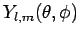, ofandare known as the spherical harmonics. Let us investigate their functional form.

Now, we know that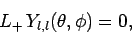(591)

since there is no state for whichhas a larger value than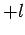. Writing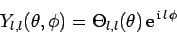(592)

[see Eqs. (570) and (574)], and making use of Eq. (555), we obtain(593)

This equation yields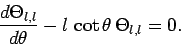(594)

which can easily be solved to give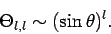(595)

Hence, we conclude that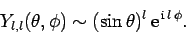(596)

Likewise, it is easy to demonstrate that(597)

Once we know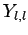, we can obtain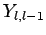by operating onwith the lowering operator. Thus,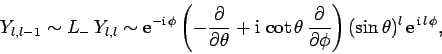(598)

where use has been made of Eq. (555). The above equation yields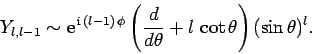(599)

Now,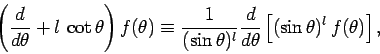(600)

where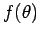is a general function. Hence, we can write(601)

Likewise, we can show that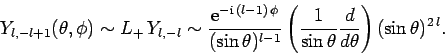(602)

We can now obtain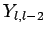by operating onwith the lowering operator. We get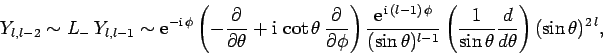(603)

which reduces to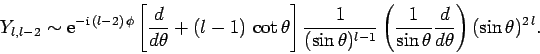(604)

Finally, making use of Eq. (600), we obtain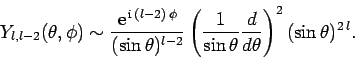(605)

Likewise, we can show that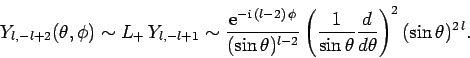(606)

A comparison of Eqs. (596), (601), and (605) reveals the general functional form of the spherical harmonics:(607)

Here,is assumed to be non-negative. Making the substitution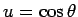, we can also write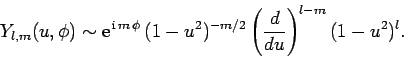(608)

Finally, it is clear from Eqs. (597), (602), and (606) that(609)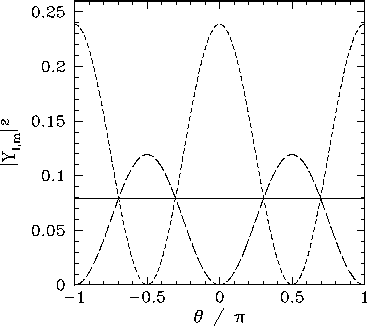We now need to normalize our spherical harmonic functions so as to ensure that(610)

After a great deal of tedious analysis, the normalized spherical harmonic functions are found to take the form(611)

for, where theare known as associated Legendre polynomials, and are written(612)

for. Alternatively,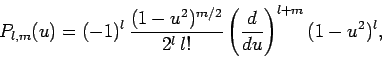(613)

for. The spherical harmonics characterized by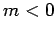can be calculated from those characterized by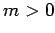via the identity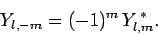(614)

The spherical harmonics are orthonormal: i.e.,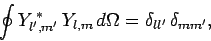(615)

and also form a complete set. In other words, any function ofand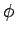can be represented as a superposition of spherical harmonics. Finally, and most importantly, the spherical harmonics are the simultaneous eigenstates ofandcorresponding to the eigenvalues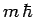and, respectively.All of the,, and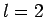spherical harmonics are listed below: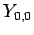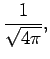(616)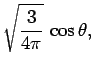(617)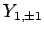(618)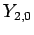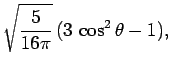(619)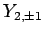(620)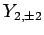(621)

Thevariation of these functions is illustrated in Figs. 18 and 19.

SubsectionsNext: Exercises Up: Orbital Angular Momentum Previous: Eigenvalues of
Richard Fitzpatrick 2010-07-20